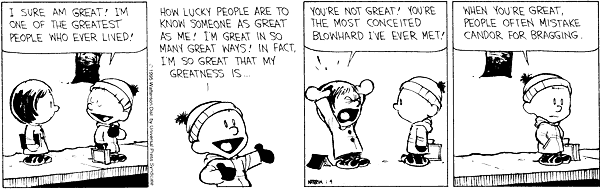# 145 Practice Test 2

1. What does the following program print out?
```public class TestExpressions{
public static void main (String[] args){
boolean a = true;
boolean b = false;
int x = 10;
int y = 5;
if (!(a && b)) {
System.out.println("Robin");}
else {
System.out.println("RedX");}
if (!(x == 10) || (y == 5)){
System.out.println("Starfire");}
else {
System.out.println("Raven");}
if (b = true && b){//tricky one
System.out.println("Cyborb");}
else {
System.out.println("BeastBoy");}

}
}

```
2. And, what does this one print out?
```public class TestLoops{
public static void main (String[] args){
boolean a = true;
boolean b = false;
int x = 10;
for (int i = 40; i > 20; i -= 4){
System.out.println(i);}
System.out.println("To infinity ");
while (x < 13) {
for (int y =x; y<13; y++){
System.out.println(y);}
x += 1;}
System.out.println("and beyond!");
}
}

```
```public class Person{
private String name;
private int year;
private double income;

public Person(String s){
this.name = s;
this.income = 0.0;
this.year = 1900;
}

public void setName(String s){
this.name = s;}

public String getName(){
return this.name;}

public double getAfterTaxIncome(){
return income * 0.9;}

public double guess(double d){
d = 500;
return 400;
}

public static void main (String[] args){
String name = "Penn";
Person p = new Person(name);
System.out.println("1-" + p.getName());
name = "Teller";
System.out.println("2-" + p.getName());
p.setName("Teller");
System.out.println("3-" + p.getName());

p.income = 10000; //10,000
System.out.println("4-" + p.income);
double income = p.getAfterTaxIncome();
System.out.println("5-" + income);
income = 500;
System.out.println("6-" + p.income);

p.income = 10000; //10,000
double d = p.guess(p.income);
System.out.println("7-" + p.income);
System.out.println("8-" + d);
}
}
```
4. Draw a UML diagram for the `Person` class shown above and for the following classes, all together.
```public class Office {
private Lawyer defense;
private Lawyer offense;
private Assistant peon1;
private Assistant peon2;
private Person worker1;
private Person worker2;
private Person worker3;
public Office() {}
}
```
```public class Assistant extends Person{
private Person boss;
public Assistant (String s, Person boss){
super(s);
this.boss = boss;}
public void makeSomeCopies (int x, String doc) {};
}
```
```public class Lawyer extends Person {
boolean passedBar;
public Lawyer (String n){
super(n);}
}
```
5. Write a short example of an interface, with two functions, and a class that implements it. Pick simple functions like `mutiplyByTwo` and `isEven`.
6. Assume you are given two global variables (I am only showing three states but assume you have all 50 states):
```Constant.acroStates = "ALAlabamaAKArkansasCACalifornia....";
Constant.acro = "ALAKCA...";
```
where `acroStates` includes all state abbreviations followed by their respective state and `acto` contains all state abbreviations in the same order as they appear in acroStates. Furthermore, you know from reading the javadocs that the following functions are defined by Java for the `String` class.
• `int indexOf(String str)` Returns the index within this string of the first occurrence of the specified substring.
• ```String substring(int beginIndex, int endIndex)``` Returns a new string that is a substring of this string. The substring begins at the specified `beginIndex` and extends to the character at index `endIndex` - 1. Thus the length of the substring is `endIndex-beginIndex`.
Write a function that, given a state's two-letter abbreviation, return's the state's full name.
7. The secret decoder ring from the Kids Next Door, Sniffling Experts Can Ruin Encoding Titillation edition, works by mapping each letter in the alphabet to another letter which is k positions after it, where k is the secret key. If that position is beyond 'z' then we wrap around to 'a'—assume only lower case letters are used. Write a function that takes as input a coded string and the secret key and prints out the decoded message.Jose M Vidal